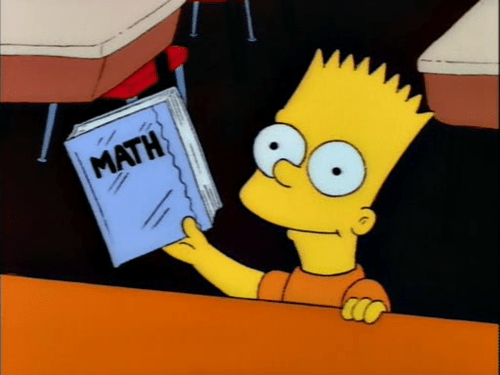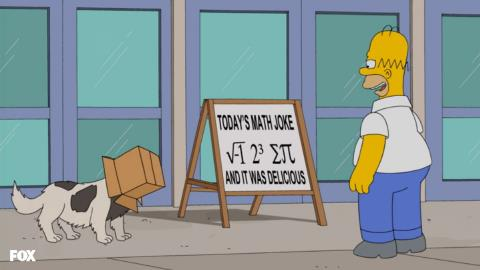# Super Safi’s Monday Morning Math Mayhem 11 – Mathlete’s Feat

Morning Mathematical Monsters & Maniacs!

(Today’s post is sponsored by the letter “M”)

Hi, I’m Super Safi and you may remember me from such stats and strategy posts as Kwik-E-Mart Farming and the advanced losing-to-win Superheroes battle strategy.

Over the past 600+ episodes, The Simpsons has taken us on an amazing mathematical journey involving fractions, probability, Fermat’s last theorem, and hundreds of other aspects from the wonderful world off mathematics.

And what better way to start your week, then by discussing math Monday morning?While celebrating Pi day last week, we looked at an epic math episode from the Season 26 finale and I left you with a math joke. This week we’ll officially break down the math joke and go through some of the math problems from that episode.

Mathlete’s Feat (Season 26, Episode 22)

When discussing π last week, I left you with a joke from Season 26, Episode 22 Mathlete’s Feat.So what does it all mean?

An imaginary number is a complex number that can be written as a real number multiplied by the imaginary unit i, which is defined by its property i2 = -1. Knowing that i2 = -1, i can be solved for as √-1. = √-1.

23 is simply the same as 2 x 2 x 2, which gives us 8.

The eighteenth letter of the Greek alphabet is sigma. Expressed as an upper case, it is Σ, and as a lowercase σ. In mathematics, the symbol Σ is used as a summation operator to calculate the sum.

And as was discussed at great length last week, the symbol π is known as Pi.

So to summarize, the board in question states:

i 8 sum Pi and it was delicious

Or if you will:

I ate some pie, and it was delicious

Ha ha ha ha ha ha ha ha ha ha ha ha ha ha ha ha ha ha ha ha ha ha ha ha ha ha ha ha ha ha ha ha ha ha ha ha ha ha ha ha ha ha ha ha ha ha ha ha ha ha ha ha ha ha ha ha ha ha ha ha ha ha ha ha ha ha ha ha ha ha ha ha ha ha ha ha ha ha ha ha ha ha ha ha ha ha ha ha ha ha ha ha ha ha ha ha ha ha ha ha ha ha ha ha ha ha ha ha ha ha ha ha ha ha ha ha ha ha ha ha ha ha ha ha ha ha ha ha ha ha ha ha ha ha ha ha ha ha ha ha ha ha ha ha ha ha ha ha ha ha ha ha ha ha ha ha ha ha ha ha ha ha ha ha ha ha ha ha ha ha ha ha ha ha ha ha ha ha ha ha ha ha ha ha ha ha ha ha ha ha ha ha ha ha ha ha ha ha ha ha ha ha ha ha ha ha ha ha ha ha ha ha ha ha ha ha ha ha ha ha ha ha ha ha ha ha ha ha ha ha ha ha ha ha ha   ow, my ribs  ha ha ha ha ha ha ha ha ha ha ha ha ha ha ha ha ha ha ha ha ha ha ha ha ha ha ha ha ha ha ha ha ha ha ha ha ha ha ha ha ha ha ha ha ha ha!

Ok, enough laughing. Now onto some serious stuff.

During the episode, the Springfield Elementary School Math team is competing against Waverly Hills Elementary’s Math team, in an event organized by Benjamin, Doug and Gary. Lets take a look at some of the questions and solve them.Question 1: What is the least common multiple of 6, 8, and 16?In mathematics, the least common multiple (also known as lowest common multiple or smallest common multiple) of two integers a and b, usually denoted by LCM(a, b), is the smallest positive integer that is divisible by both a and b. The least common multiple of three integers ab, and c, usually denoted by LCM(a, b, c), is the smallest positive integer that is divisible by ab, and c.

So to solve for the LCM(6,8,16), let’s look at the multiples of 6, 8, and 16:

6 – 6, 12, 18, 24, 30, 36, 42, 48, 54, 60

8 – 8, 16, 24, 32, 40, 48, 56, 64, 72, 80

16 – 16, 32, 48, 64, 80, 96, 112, 128, 144, 160

As you can see, 48 is the least common multiple, as it is the smallest number that is divisible by 6, 8, and 16.

While LCM(6,8,16) is 48, let’s just take a few seconds to appreciate LCM(6,8) is 24, LCM(6,16) is also 48, and LCM(8,16) is 16.

Question 2: What is the value of (3+52) / 4 – (x+1) when x = 7?In mathematics, the order of operations is a collection of rules that reflect conventions about which procedures to perform first in order to evaluate a given mathematical expression. The order of operations is as follows:

1. Bracket/parenthesis
2. Exponents and roots
3. Multiplication and division
4. Addition and subtraction

Mnemonics used to memorize the order include:

• BEDMAS – Bracket, exponent, division, multiplication, addition, subtraction
• PEDMAS – Parenthèsis, exposants, division, multiplication, addition, soustraction
• PEMDAS – Parenthesis, exponent, multiplication, division, addition, subtraction
• BIDMAS – Bracket, indices, division, multiplication, addition, subtraction
• BODMAS – Bracket, order, division, multiplication, addition, subtraction

(3+52) / 4 – (x+1)   =  (3+52) / 4 – (7+1)   =   (55)  / 4 – (8)   =   13.75 – 8   =   5.75

Question 3: If x + 4y = 5 and 5x + 6y = 7, then 3x + 5y = ?Thanks to reader JMP for the correction: If x + 4y = 5 and 5x + 6y = 7, we can add both equations together to get x + 4y + 5x + 6y = 5 + 7, or 6x + 10y = 12. If we divide this by 2, we get 3x + 5y = 6.

48, 5.75, 6! Woohoo! We did it. Here’s our Award:Now are you ready to go join your local mathlete team and compete in the mathlympics? Did you remember this episodes? Were you able to solve the problems on your own? Sound off in the comments below. You know we love hearing from you.

### 10 responses to “Super Safi’s Monday Morning Math Mayhem 11 – Mathlete’s Feat”

1.stewie22112

I’m always slightly intrigued by those relatively simply BODMAS (Oz, 1980’s) posts on Facebook. Or more specifically, the sheer volume of wrong answers posted in the comments.

I hope they’re bots and not real people

2.rudolphovanwijk

The order of signs have been changed quite often during the history of mathematics
As a rule of thumb I’ve learned “Meneer Van Dalen Wacht Op Antwoord” (Mister van Dalen waits for answer)
1. Machtsverheffen (power)
2. Vermenigvuldigen (multiply)
3. Delen (division)
4. Worteltrekken (root)
5. Optellen (Adding)/Aftrekken (subtraction)
It is also the order of calculation.
12 : 4 × 3 is 1 in this way (It is should be 9)
√ 4 × 3 was √(12), now it is 6
Parentheses were thought later.

3.Mr.Black

Once you learn BEDMAS (i like to sleep more) the rest is a piece of cake!
~MIB👤

•Safi

LOL

4.rudolphovanwijk

There’s a curve for matlete’s foot, making moda

•rudolphovanwijk

And of course marlic.

5.koolviruz

holy molly I don’t think I remember this episode… by the looks of it Bart is smarter than me.. I couldn’t solve any of them… maybe I been out of school for so long that I don’t remember. or it could be that I deal mostly with 0’s and 1’s…

6.Tracy-1ltwoody920

I did not get the math.
But speaking of pie

🎶🎶🎶🎶

Blind Boy Fuller sang in 12 Bar Blues format, circa 1939
🎶🎶🎶🎶🎶
I’m not joking I’ll tell you no lie. I want some of your custard pie
You gotta give me some of it. You gotta give me some of it
You gotta give me some of it, before you give it all away
🎶🎶🎶🎶🎶
Hank Williams later sang Move it on Over to the same tune.

7.JMP

Ehm, your solution to the last one is wrong. You actually don’t need to solve the system at all. Add both input equation, and you get 6x+10y = 12. Divide by 2 => 3x+5y=6. End of solution.

8.LucaSpadazzi

Thanks a lot for this post Safi! I also competed in a mathlympics, 17 years ago, when I was a young promise XD